# Cyclic voltammetry

## Introduction​

Cyclic voltammetry is a type of potentiodynamic electrochemical measurement. A cyclic voltammetry graph is a graph of the current as a function of the potential. Both of these variables depend on time.

This tool allows the importation of data as a txt or a JCAMP file.

In this view, it is possible to upload one or more spectra of a target sample by drag/drop either a JCAMP-DX or a text file. An individual spectrum can be visualized and analyzed by clicking on its name in the "List of files". Several spectra can also be compared. The information about the chosen sample can be found in the colored window on the right.

You can upload a spectrum in 3 different ways:

• by dragging your files and then dropping them in the designated zone.
• by clicking on the upload zone and selecting the files to upload.
• by copying the spectrum into the clipboard and then pasting it using CTRL+V (or ⌘+V on MacOS).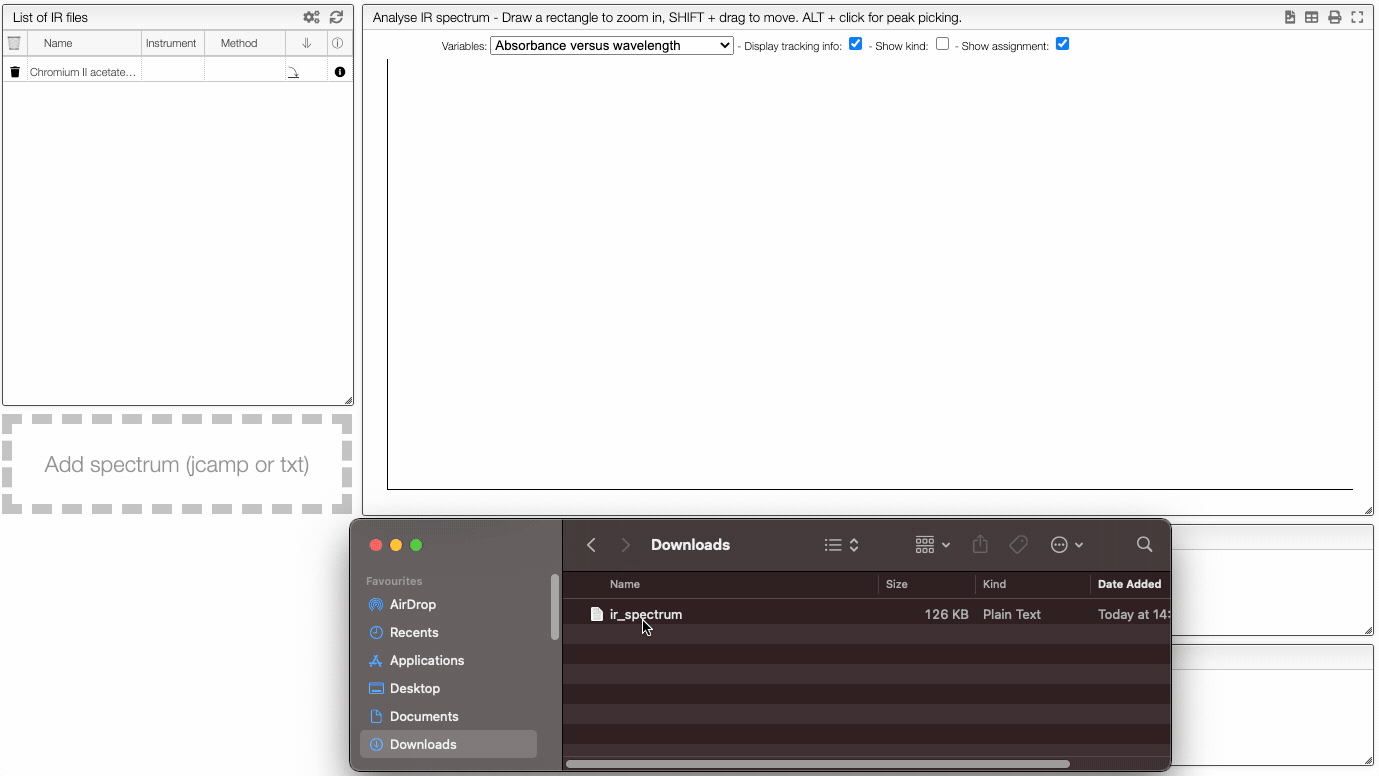The format of the file to be uploaded should be JCAMP-DX (extension .dx or .jdx), this is the usual format used on most IR spectrophotometers.

If you click on an imported file, the spectrum is plotted on the middle panel as following. You can manipulate it.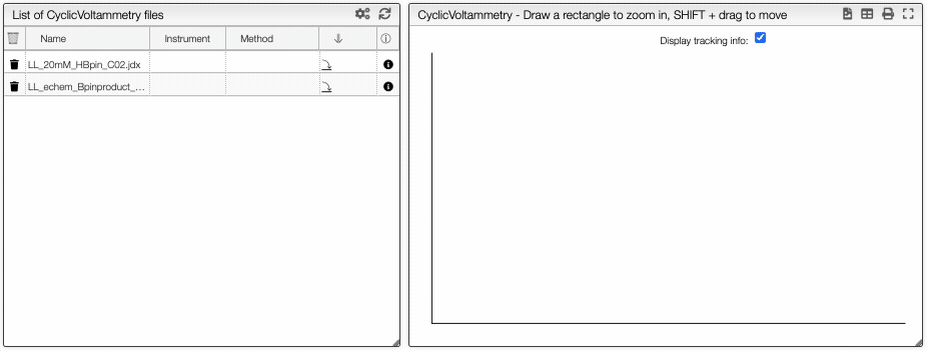## Preprocessing​

On the right panel, you can apply mathematical operations to the spectra with the preprocessing module.

Spectrum preprocessing
Apply mathematical tools to the spectra.

## Preprocessing​

You can apply the following modifications to the spectra to enhance the visualization. The modifications include the following:

• Center Mean : subtract the mean from every variable observation in the dataset, so that the new variable's mean is centered at 0.
• Divide by SD : divide every variable observable in the dataset by the standard deviation yields a distribution with a standard deviation equal to 1.
• Divide by max Y : divide every value by the maximum y-value shifts all the y-values between 0 and 1.
• Rescale (x to y) : rescale the graph such that the y-values fit between specified minimum and maximum values.
• Normalize (sum to n) : normalize the integral under the curve so that it sums to n.
• Multiply (value) : multiply every y-value by a scalar.
• Add (value) : add a scalar to every y-value.
• AirPLS baseline : baseline correction using adaptive iterative reweighed penalized least squares algorithm.
• Rolling average baseline :
• Iterative polynomial baseline : baseline correction using iterative polynomial fitting algorithm.
• Rolling ball baseline :
• Rolling median baseline :

A certain range of x-values can be selected to show only a part of the spectrum using Range.

Depending on the analysis, some regions should be removed using Exclusions in order to improve the visualization.## Compare spectra​

You can compare multiple spectrum by clickling on the Compare button. It is then possible to hide/show spectra.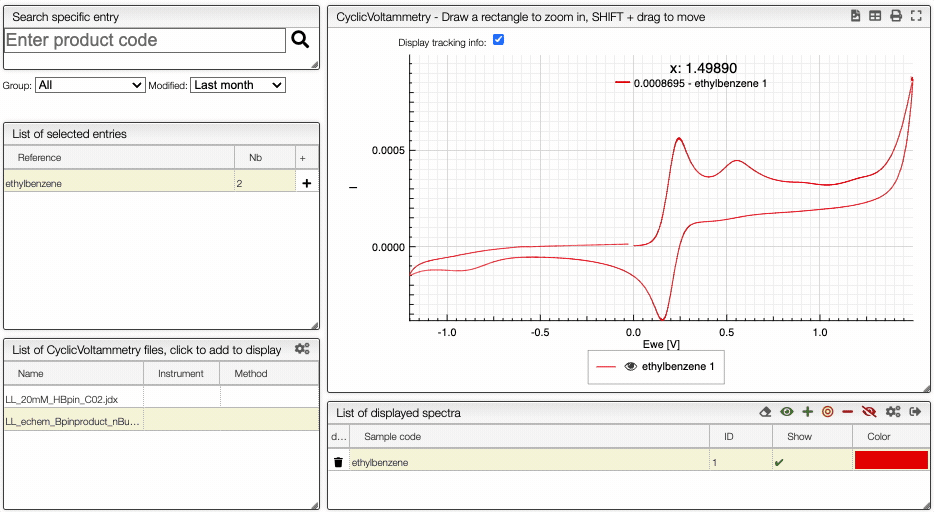Columns customization
In many tables it is possible to select which columns to display. This is achieved by clicking on the icon.

After clicking on the icon a dialog box opens that allows to add a new column.

There are 5 parameters to fill for a new column:

• name: the column name. This will be displayed as the header to the column.
• rendererOptions: options that allows among other to format numbers. One very useful formatter is numeral (see after)
• width: number of pixels for the specific column. May stay empty for automatic layout.
• forceType: select how to display complex values (see later)
• jpath: where to find the information to display in the column

In order to add a new column you need to select the jpath using the hierarchical drop down menu.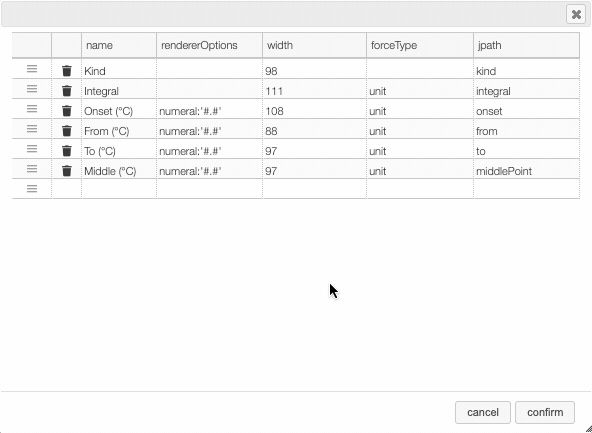Columns can be moved or rearranged as well.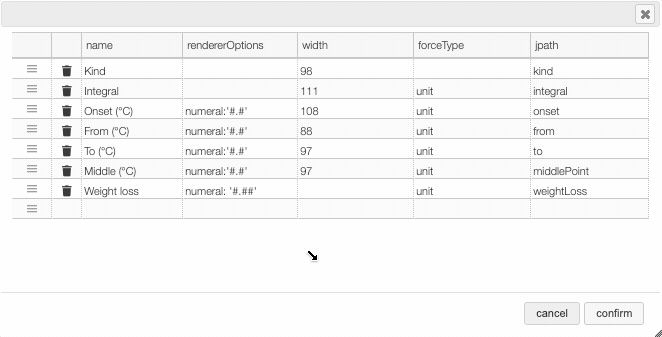rendererOptions: numeral

number,

Value to formatrendererOptionsResult
12.345678numeral:'#.##'12.34
12.3numeral:'#.00'12.30
0.3numeral:'#.0 %'30.0 %

forceType

In the database some values are stored as an object that needs to be displayed to the user in an intuitive way.

For example the unit type will store in the database the value using as units SI (we convert the data to the units defined by the 'standard international') and specify in which units the user would like to display the data. So in the following example we are in fact storing the value -150°C by storing the value in SI (Kelvin) and specifying that the user wants to see it in °C.

{  "SI": 123.15,  "unit": "°C"}

Another example is the valueUnits type that will store the data in 2 different properties (value and unit). In this case the value is stored in the specified units and there is no conversions.

{  "value": 123,  "units": "°C"}

While this way to store the data in the database is very practical it is not the way that the user would like to see his results. We have therefore the possibility to forceType: define how the user would like to see the results.

Other types include mf. This formatter allows to correctly display a molecular formula that is stored in the database as "C10H20O3". i.e. it will put the numbers in subscript (C₁₀H₂₀O₃).